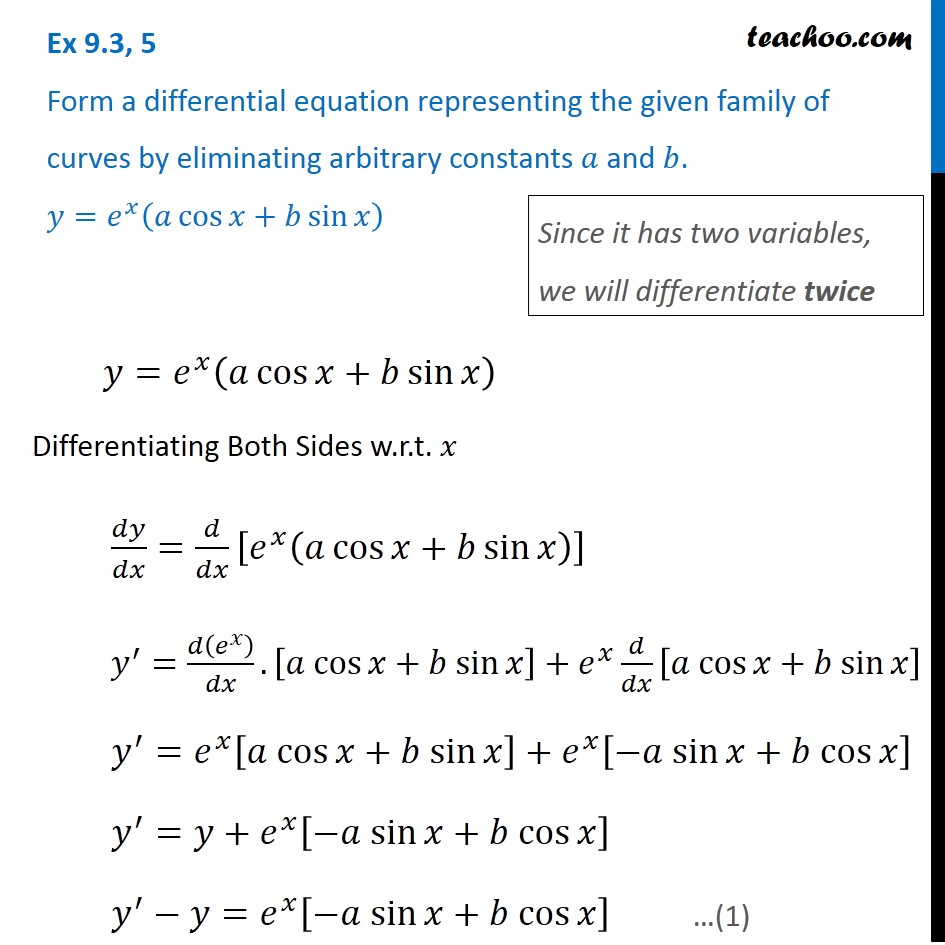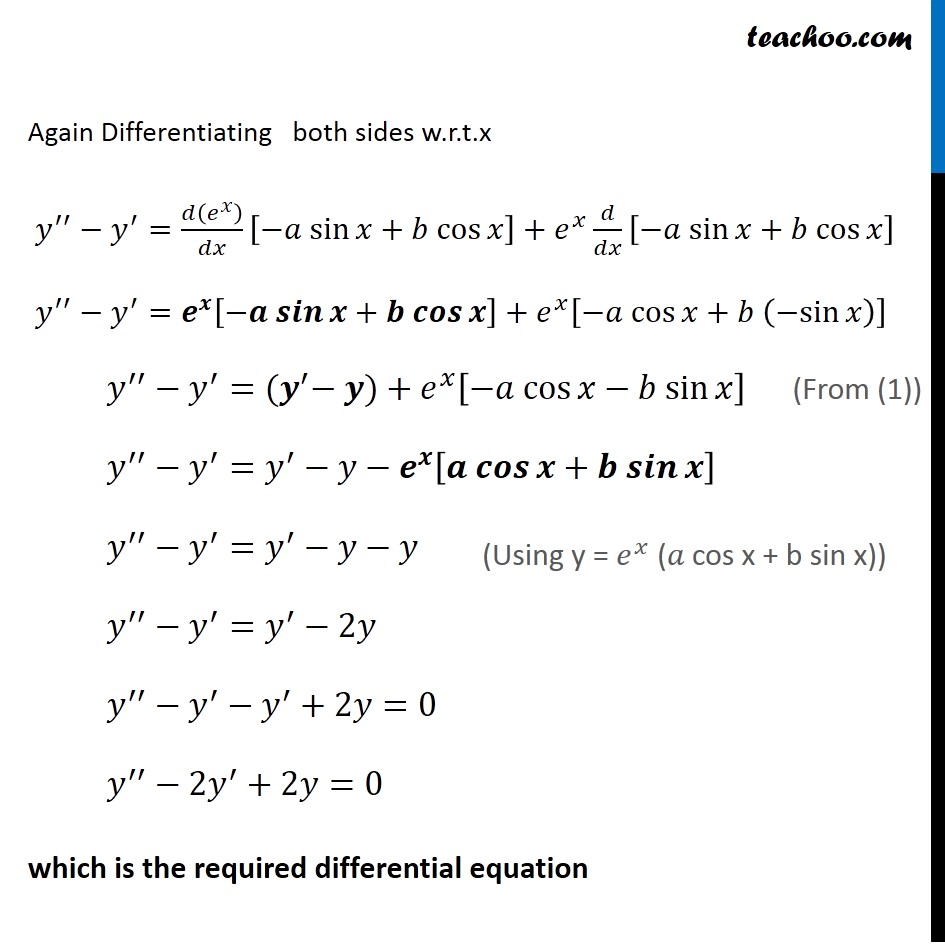Forming Differential equations

Chapter 9 Class 12 Differential Equations
Serial order wiseLearn in your speed, with individual attention - Teachoo Maths 1-on-1 Class

### Transcript

Question 5 Form a differential equation representing the given family of curves by eliminating arbitrary constants 𝑎 and 𝑏. 𝑦=𝑒^𝑥 (𝑎 cos⁡〖𝑥+𝑏 sin⁡𝑥 〗 ) Since it has two variables, we will differentiate twice 𝑦=𝑒^𝑥 (𝑎 cos⁡〖𝑥+𝑏 sin⁡𝑥 〗 ) Differentiating Both Sides w.r.t. 𝑥 𝑑𝑦/𝑑𝑥=𝑑/𝑑𝑥 [𝑒^𝑥 (𝑎 cos⁡𝑥+𝑏 sin⁡𝑥 )] 𝑦^′=𝑑(𝑒^𝑥 )/𝑑𝑥.[𝑎 cos⁡𝑥+𝑏 sin⁡𝑥]+𝑒^𝑥 𝑑/𝑑𝑥 [𝑎 cos⁡𝑥+𝑏 sin⁡𝑥] 𝑦^′=𝑒^𝑥 [𝑎 cos⁡𝑥+𝑏 sin⁡𝑥]+𝑒^𝑥 [−𝑎 sin⁡𝑥+𝑏 cos⁡𝑥] 𝑦^′=𝑦+𝑒^𝑥 [−𝑎 sin⁡𝑥+𝑏 cos⁡𝑥] 𝑦^′−𝑦=𝑒^𝑥 [−𝑎 sin⁡𝑥+𝑏 cos⁡𝑥] …(1) Again Differentiating both sides w.r.t.x 𝑦^′′−𝑦^′=𝑑(𝑒^𝑥 )/𝑑𝑥 [−𝑎 sin⁡𝑥+𝑏 cos⁡𝑥]+𝑒^𝑥 𝑑/𝑑𝑥 [−𝑎 sin⁡𝑥+𝑏 cos⁡𝑥] 𝑦^′′−𝑦^′=𝒆^𝒙 [−𝒂 𝒔𝒊𝒏⁡𝒙+𝒃 𝒄𝒐𝒔⁡𝒙]+𝑒^𝑥 [−𝑎 cos⁡𝑥+𝑏 (−sin⁡𝑥)] 𝑦^′′−𝑦^′=〖(𝒚〗^′− 𝒚)+𝑒^𝑥 [−𝑎 cos⁡𝑥−𝑏 sin⁡𝑥] 𝑦^′′−𝑦^′=𝑦^′−𝑦−𝒆^𝒙 [𝒂 𝒄𝒐𝒔⁡𝒙+𝒃 𝒔𝒊𝒏⁡𝒙] 𝑦^′′−𝑦^′=𝑦^′−𝑦−𝑦 𝑦^′′−𝑦^′=𝑦^′−2𝑦 𝑦^′′−𝑦^′−𝑦^′+2𝑦=0 𝑦^′′−2𝑦^′+2𝑦=0 which is the required differential equation (From (1)) (Using y = 𝑒^𝑥 (𝑎 cos x + b sin x))